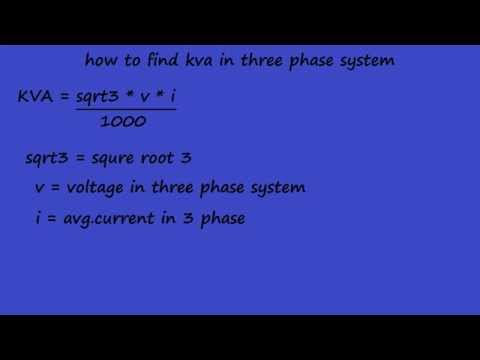### Main Difference

KVA and KW are the terms related to electrical systems. Both are units of electrical power. Electrical power is distributed in three types i.e. real power, apparent power, and reactive power. KW is real power that actually does work. KVA is the apparent power and only a small fraction of it does work. In DC the KVA and KW are equal while in AC system the KVA and KW are different from each other. The difference between KVA and KW is due to the phase difference between current and voltage varying power factor from unity either leading or lagging.

### What is KVA?

It is termed as kilo Volts Ampere. KVA is the apparent power and only a small fraction of it does work. Mathematically the relation of KVA with KW is kVA = kW/power factor.

### What is KW?

It is termed as kilo Watts. KW is real power that actually does work. Mathematically the relation of KW with KVA is kW = kVA x power factor. The real power of an AC system depends upon the power factor which is cos@.

### Key Differences

1. KW is the real electrical power while KVA is the apparent electrical power.
2. KW is termed as kilo Watts while KVA is termed as kilo Volta Amperes.
3. KW is the amount of work done by electricity while only a small fraction of KVA does work.
4. Electrical appliances dealing with power factor are rated in KW while those not dealing with power factor are rated in KVA.
5. Transformers are rated in KVA while generators are rated in KW.
6. KVA and KW are equal in DC system while they differ in AC system.
7. The difference between KVA and KW occurs due to the phase difference between voltage and current.
8. The mathematical equation for KVA is kVA = kW / PF while KW is kW = kVA x PF.